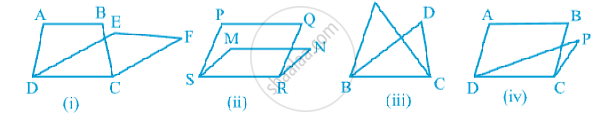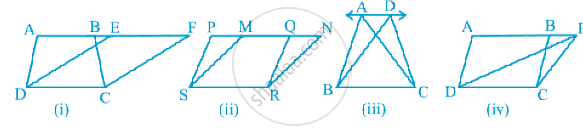Share

# Figures on the Same Base and Between the Same Parallels

Course

#### notes

Look at the following figures:In Fig. (i), trapezium ABCD and parallelogram EFCD have a common side DC. We say that trapezium ABCD and parallelogram EFCD are on the same base DC.
Similarly, in Fig.(ii), parallelograms PQRS and MNRS are on the same base SR;
In Fig.(iii), triangles ABC and DBC are on the same base BC and in Fig.(iv), parallelogram ABCD and triangle PDC are on the same base DC.
Now look at the following figures:In Fig. (i), clearly trapezium ABCD and parallelogram EFCD are on the same base DC. In addition to the above, the vertices A and B (of trapezium ABCD) opposite to  base DC and the vertices E and F (of parallelogram EFCD) opposite to base DC lie on a line AF parallel to DC. We say that trapezium ABCD and parallelogram EFCD are on the same base DC and between the same parallels AF and DC. Similarly, parallelograms PQRS and MNRS are on the same base SR and between the same parallels PN and SR [see Fig.(ii)] as vertices P and Q of PQRS and vertices M and N of MNRS lie on a line PN parallel to base SR.In the same way, triangles ABC and DBC lie on the same base BC and between the same parallels AD and  BC [see Fig. (iii)] and parallelogram ABCD and triangle PCD lie on the same base DC and between the same parallels AP and DC [see Fig.(iv)].

So, two figures are said to be on the same base and between the same parallels, if they have  a common base (side) and the vertices (or the vertex) opposite to the common base of each figure lie on a line parallel to the base.

### Shaalaa.com

Figures on the same base and betwee the same parallels [00:18:06]
S
0%

S